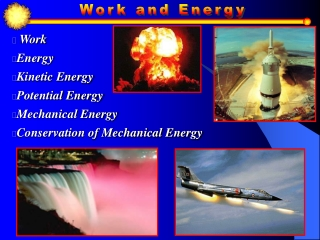DownloadDownload PresentationWork Energy Kinetic Energy Potential Energy Mechanical Energy Conservation of Mechanical Energy

# Work Energy Kinetic Energy Potential Energy Mechanical Energy Conservation of Mechanical Energy

Download Presentation## Work Energy Kinetic Energy Potential Energy Mechanical Energy Conservation of Mechanical Energy

- - - - - - - - - - - - - - - - - - - - - - - - - - - E N D - - - - - - - - - - - - - - - - - - - - - - - - - - -
##### Presentation Transcript

1. Work • Energy • Kinetic Energy • Potential Energy • Mechanical Energy • Conservation of Mechanical Energy

2. ENERGY • The capacity to do work • The energy transferred to an object equals the work done on the object  ET = W

3. Energy is present in many forms • Heat (thermal energy) • Kinetic Energy = “Motion Energy” • Potential Energy = “Stored Energy” • Mechanical energy – Sum of Potential and Kinetic • Nuclear • Sound • Electromagnetic energy • Chemical energy

4. The parallel component does work The (F cos ) component does work

5. NO work done if there: • Is no movement • The force if perpendicular to the displacement

6. In which photo(s) is WORKbeing done? no yes yes no

7. If a 10 Newton force displaces a 20 kg block 40 meters calculate the work done on the block by the force. F = 10N EXAMPLE The Normal force (FN ) and weight (mg) do no work in this problem, WHY?

8. Work = Force  Distance • The component of force parallel to the displacement DOES WORK • The perpendicular component DOES NO WORK •  - Between the force and displacement • Scalar • Measured in Joules (J)

9. Positive Work - Force and displacement in same direction. Negative Work - Force opposite the displacement. Zero Work - Force is perpendicular to the displacement. F d F d F d

10. If the Force is in the Direction of Motion: In this case,  = 0 o, so:                  If the Force is in the Opposite Direction of Motion: In this case,  = 180o, so:

11. If the Force is Perpendicular to the Direction of Motion: In this case,  = 90 o, so:                 If the Object Being Pushed Doesn't Move: In this case, x = 0, so:

12. F= 10 N 300 A 10 N force acts 300 above the horizontal and displaces an object 5 meters horizontally how much work is done? W= F d cos  = (10N)(5m) cos 300 = 43.3 J

13. How much work does gravity do on a 70 kg person who falls 100 meters in the free fall ride?

14. Kinetic energy (KE) is the energy of a moving object. • Energy associated with motion and mass.

15. A 500 kg car is traveling at 10 m/s, 10 sec. later it is traveling 30 m/s. Calculate the following: • The initial kinetic energy • The final kinetic energy • The change in KE

16. The animation shows a block of mass and initial speed v sliding across a floor that is not frictionless. A kinetic friction force fk stops block during displacement d. Thus we can relate work done by friction to the change E in the system's energy

17. For hammer: Moving hammer can do work on nail! For nail:

18. Matt’s little red wagon with a mass of 4.6 kg moves in a straight line • on a frictionless horizontal surface. It has an initial speed of 10 m/s • and is pulled by Matt 4.0 m with a force of 18N in the direction of • the initial velocity. Use the work-energy relation • (WNet = KE) to calculate the wagons: • Change in Kinetic Energy • Final speed.

19. In a test of old sports car, it’s found that engines provided around 1,000 N of force. If the typical mass is 400 kg and they accelerate from rest, how fast will they be going 100 m down the road?

20. How much work is done holding a box in place on an incline? How much work is done pushing a 15 kg box up a 30° incline at a constant speed for 3 m

21. Force vs. Distance Area under curve equals the work done

22. Force vs. Distance Area under curve equals the work done

23. Area above the curve – work is positive Area below the curve – work is negative

24. Work done by a variable force equals Area under the curve

25. Gravitational Potential Energy (PE): The energy an object has due to its height above a reference point. The potential energy change is independent of the path between the initial and final points. link

26. PE=mgh Can gains Potential Energy equal to mgh

27. One serving of Bagel Crisps contains 543 kJ. How many pull-ups are needed to burn it off? • M = 60 kg ∆h = .5 m • ∆PE = mg ∆H • 543000J = (60kg)(9.81N/kg)(.5m)(n) • 1845 = n • But the human body is only abour 20% efficient so, n is only 369!

28. Who does more work in lifting the respective equal masses to the top of the incline at a constant speed?

29. 1. His initial potential energy with respect to the ground 2. His potential energy 1 seconds after being released 3. His change in potential energy 4. Where did its energy go when it hits the ground? Rufus the 5 kg cat falls 10 meters from above the surface of the earth. Calculate:

30. Conservative Force: The work done is independent of the path taken. Only depends on the initial and final position. Ex: Gravity Non Conservative Force: The work done depends on the path taken. Ex: Friction

31. Don’t disregard the Conservation Laws